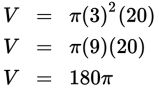# SAT Math Multiple Choice Question 477: Answer and Explanation

### Test Information

Question: 477

12. In geology, the water table is the level below which the ground is saturated with water. Wells must be dug below this point to bring water up into the well. Except in cases of severe flooding, the water level in a well does not rise above the water table. Suppose a cylindrical well is 6 feet wide and 60 feet deep in an area where the water table is 40 feet below ground level. Assuming no unusual circumstances, what is the volume in cubic feet of the water in the well at any given time?

• A. 180π
• B. 360π
• C. 540π
• D. 720π

Explanation:

A

Difficulty: Hard

Category: Additional Topics in Math / Geometry

Strategic Advice: Use the formula for finding the volume of a cylinder, V = πr2h. Check the formula page rather than trying to recall it from memory. Don't forget to use the radius, not the diameter, which is given.

Getting to the Answer: The well is 6 feet wide; this is its diameter, so r = 3. The height of the well is 60 feet, but the water table is 40 feet below ground level, which means only 60 – 40 = 20 feet of the well is below the water table and thus has water in it, so h = 20.